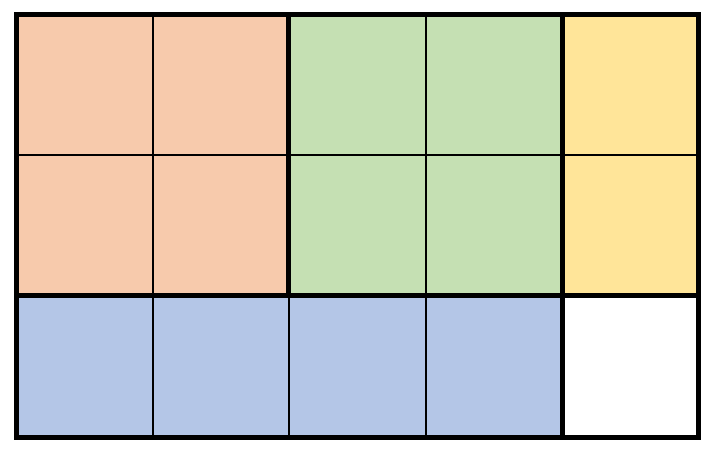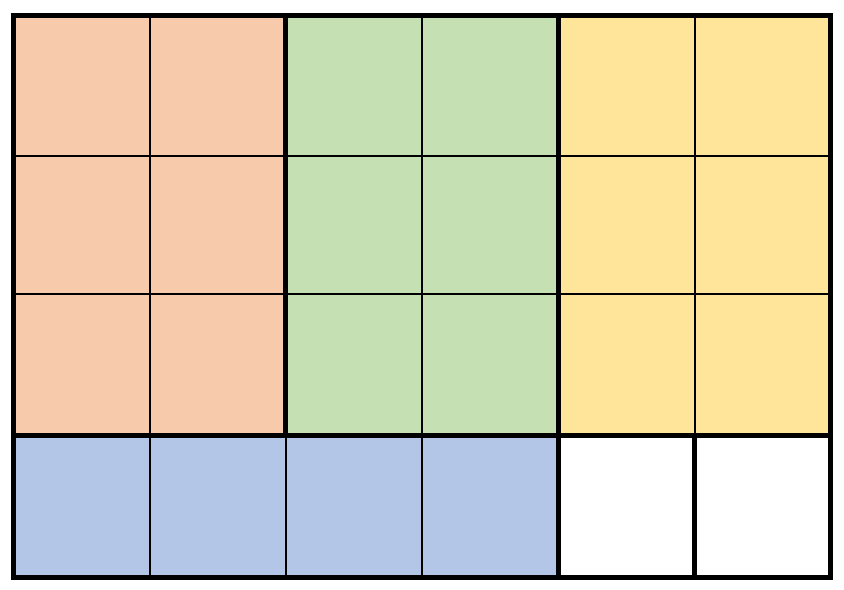## 战局详情

844 / 6228Juruoer121:07:070:10:160:27:01 11:02:07

## 题目及解答

5242. 兼具大小写的最好英文字母

• 1 <= s.length <= 1000
• s 由小写和大写英文字母组成

5218. 个位数字为 K 的整数之和

• 每个整数个位数字都是 k
• 所有整数之和是 num

• 多重集与集合类似，但多重集可以包含多个同一整数，空多重集的和为 0 。
• 个位数字 是数字最右边的数位。

• 0 <= num <= 3000
• 0 <= k <= 9

6099. 小于等于 K 的最长二进制子序列

• 子序列可以有 前导 0
• 空字符串视为 0
• 子序列 是指从一个字符串中删除零个或者多个字符后，不改变顺序得到的剩余字符序列。

• 1 <= s.length <= 1000
• s[i] 要么是 '0' ，要么是 '1'
• 1 <= k <= 1e9

s 的长度为 nk 的二进制（首位为 1）的长度为 m

• n <= m ，则 s 的所有后缀的二进制均小于等于 k，返回 n。
• n > m，且 s 的长为 m 的后缀的二进制小于等于 k，返回 s 中除去长为 m 的后的部分的 0 的个数 + m
• n > m，且 s 的成为 m 的后缀的二进制大于 k，说明 s 的长为 m 的后缀以 '1' 开头，又 s 的长为 m - 1 的后缀的二进制必小于 k，故返回 s 中除去长为 m 的后的部分的 0 的个数 + m - 1

5254. 卖木头块

• 沿垂直方向按高度 完全 切割木块，或
• 沿水平方向按宽度 完全 切割木块

• 1 <= m, n <= 200
• 1 <= prices.length <= 2 * 1e4
• prices[i].length == 3
• 1 <= hi <= m
• 1 <= wi <= n
• 1 <= pricei <= 1e6
• 所有 (hi, wi) 互不相同 。• 2 块 2 x 2 的小木块，售出 2 * 7 = 14 元。
• 1 块 2 x 1 的小木块，售出 1 * 3 = 3 元。
• 1 块 1 x 4 的小木块，售出 1 * 2 = 2 元。

19 元是最多能得到的钱数。• 3 块 3 x 2 的小木块，售出 3 * 10 = 30 元。
• 1 块 1 x 4 的小木块，售出 1 * 2 = 2 元。

32 元是最多能得到的钱数。

• 高度为 i ，宽度分别为 kj - k 的两块，其中 k 大于 0 且 小于 j，或是
• 宽度为 j ， 高度分别为 ki - k 的两块，其中 k 大于 0 且 小于 i

dp[i][j] = max(max(dp[i][k] + dp[i][j - k]), max(dp[k][j] + dp[i - k][j]))